0
2635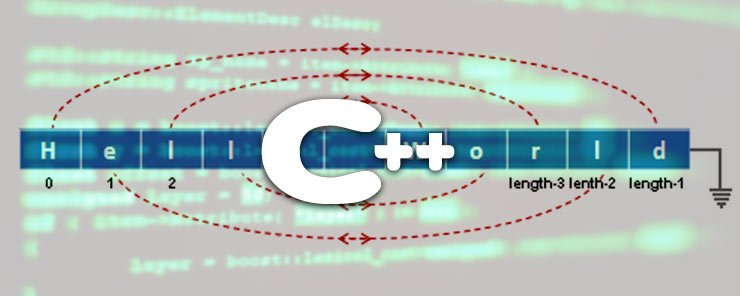In the previous two articles, we introduced strings. Then, we learned some of the useful functions that C++ offers to manipulate strings. In this article, we are going to continue with the string functions. Have a nice reading!

The strrev() Function
This is the string reverse function. It reverses a given string by reversing the order of all characters. As with other string functions, the string.h library file must be included.

Example
Let’s see how this function works by using an example.

```#include <iostream.h>
#include <conio.h>
#include <string.h>
void main()
{
clrscr();
char greeting[] = "Aloha";
cout << "\nOriginal String:\t" << greeting << endl;
strrev(greeting);
cout << "\nReversed String:\t" << greeting;
getch();
}
```

When executed, the program should give the following output: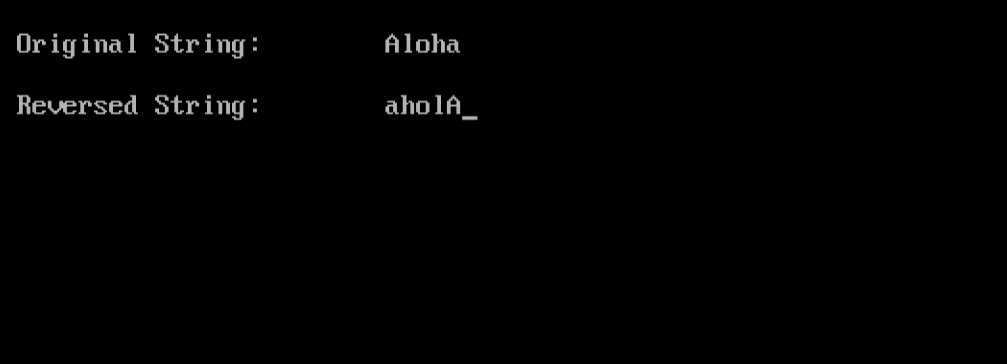Notice that the original string has been reversed; i.e. the string variable greeting has its string reversed.

strlwr() and strupr() Functions
The strlwr() function converts all the letters in a string to lowercase letters. Conversely, the strupr() function converts all to uppercase. Both functions change the original string variable, and both require including the string.h header file.

Example
The following program converts the letters of the name of my favorite Italian footballer “Alessandro Del Piero” into lowercase, then uppercase, and prints both forms.

```#include <iostream.h>
#include <conio.h>
#include <string.h>
void main()
{
clrscr();
char player[] = "Alessandro DEL PIERO";
cout << "\nOriginal String:\t" << player << endl;
strlwr(player);
cout << "\nLowercase String:\t" << player;
strupr(player);
cout << "\nUppercase String:\t" << player;
getch();
}
```

The program should print the following output: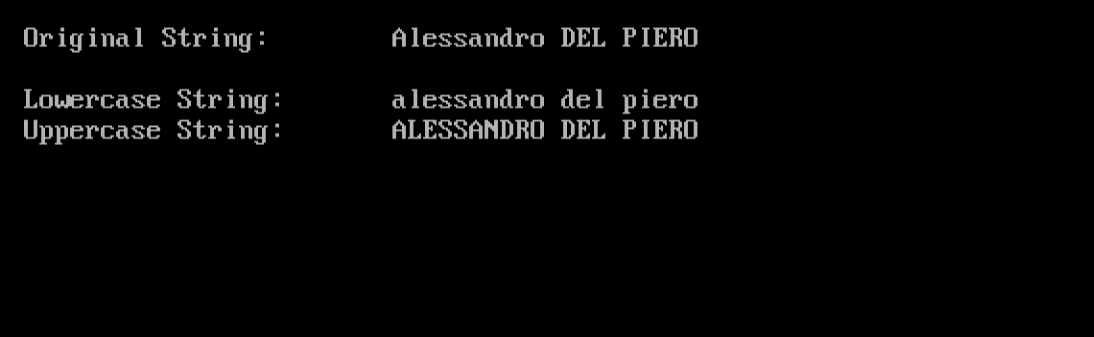Interesting!

The strset() Function
This function sets all characters in a string to a given character.

Syntax

`strset(STRINGVAR, CH);`

Where:

STRINGVAR is a string variable.
CH is the character to set all characters in STRINGVAR to.

Example
The following program sets all characters in the string str to ‘=’

```#include <iostream.h>
#include <conio.h>
#include <string.h>
void main()
{
clrscr();
char str[] = "abcdefghijk";
cout << "\nOriginal String:\t" << str << endl;
strset(str,'=');
cout << "\nConverted String:\t" << str;
getch();
}
```

Executing this code should print the following output: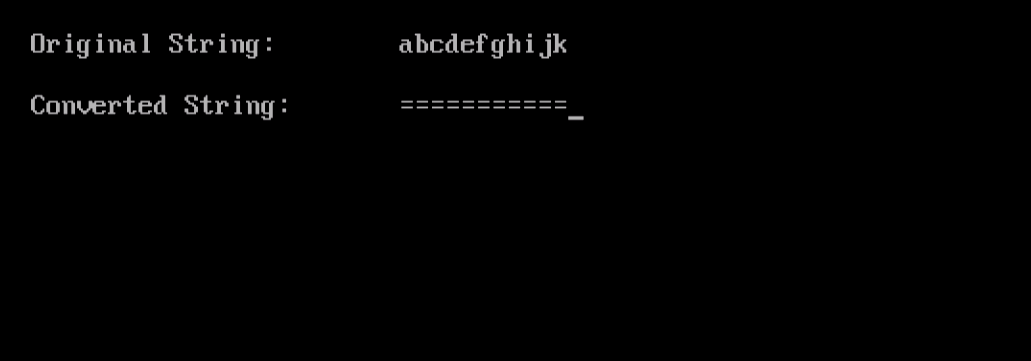The strstr() Function
This function searches a string for the first occurrence of another sub-string. It returns a pointer (for now, consider it as an address) to the first occurrence of the sub-string , or null if the sub-string is not found.

Example
The following program searches for the first occurrence of the string “ro” within the name of the Italian superstar “Alessandro Del Piero”.

```#include <conio.h>
#include <iostream.h>
#include <string.h>
int main()
{
clrscr();
char superstr[] = "Alessandro Del Piero";
char substr[] = "ro";
char *ptr;
ptr = strstr(superstr, substr);
cout << endl << ptr;
getch();
return 0;
}
```

When executed, the program should print the part of the superstring where the sub-string “ro” was first matched.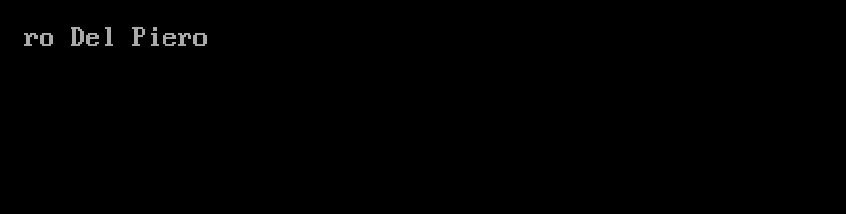One thing you will find different in this program is the declaration of the main() function.

```int main()
and the last line:
return 0;
```

The main() function is like any function; it can take arguments, and return values. In all the previous programs we have written so far in this series, we have always specified void as the return type of the main(), i.e. it doesn’t return anything. In this program, we have specified int as the type of the returned value, so the function has to return an integer number. The main() function will return this integer to the program or the operating system that invoked it. This is very useful to report back the exit status of the main(); i.e. the program started by the main() function. If you are a UNIX/Linux user, you would know about the exit status of commands and scripts.

The string Class
Fortunately, C++ was nice enough to introduce new standard class to implement strings. The string class handles all the tasks of sizing and memory management on behalf for you! In addition, it offers a set of useful functions and operators that facilitate many of your needs. Isn’t it great?

The string class is considered as a lately-developed feature (which was introduced starting with C++ 98 in 1998). As a result, the old C++ compilers don’t support this class. For this reason, we are going to install another new IDE for developing, compiling and executing C++ programs. OK, let’s do it!

Installing Dev-C++
Dev-C++ is free IDE software for developing C and C++ programs. To download Dev-C++, open your Internet browser, and navigate to the following URL: http://sourceforge.net/projects/orwelldevcpp/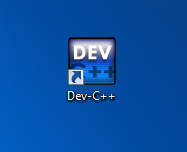Congratulations!

Summary